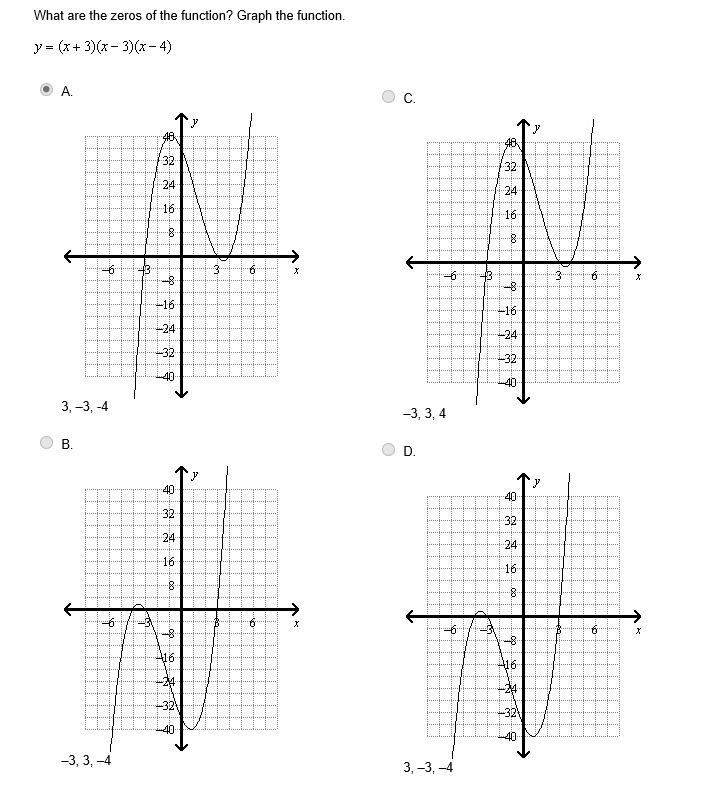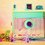I think the answer is C but i'm not 100% sure!Note by Christina Morris
6 years, 9 months ago

This discussion board is a place to discuss our Daily Challenges and the math and science related to those challenges. Explanations are more than just a solution — they should explain the steps and thinking strategies that you used to obtain the solution. Comments should further the discussion of math and science.

When posting on Brilliant:

• Use the emojis to react to an explanation, whether you're congratulating a job well done , or just really confused .
• Ask specific questions about the challenge or the steps in somebody's explanation. Well-posed questions can add a lot to the discussion, but posting "I don't understand!" doesn't help anyone.
• Try to contribute something new to the discussion, whether it is an extension, generalization or other idea related to the challenge.

MarkdownAppears as
*italics* or _italics_ italics
**bold** or __bold__ bold
- bulleted- list
• bulleted
• list
1. numbered2. list
1. numbered
2. list
Note: you must add a full line of space before and after lists for them to show up correctly
paragraph 1paragraph 2

paragraph 1

paragraph 2

[example link](https://brilliant.org)example link
> This is a quote
This is a quote
    # I indented these lines
# 4 spaces, and now they show
# up as a code block.

print "hello world"
# I indented these lines
# 4 spaces, and now they show
# up as a code block.

print "hello world"
MathAppears as
Remember to wrap math in $$ ... $$ or $ ... $ to ensure proper formatting.
2 \times 3 $2 \times 3$
2^{34} $2^{34}$
a_{i-1} $a_{i-1}$
\frac{2}{3} $\frac{2}{3}$
\sqrt{2} $\sqrt{2}$
\sum_{i=1}^3 $\sum_{i=1}^3$
\sin \theta $\sin \theta$
\boxed{123} $\boxed{123}$

Sort by:

The answer is C. Set each factor of the formula of the function equal to 0 and solve for x in order to find the x-intercepts: x + 3 = 0 then x = - 3; x - 3 = 0 then x = 3; x - 4 =0 then x = 4. Therefore, the graph of the function has x-intercepts - 3, 3 and 4.

- 6 years, 9 months ago

A has the same solutions...Why not A??

- 6 years, 9 months ago

Because the zeros in A) are not in ascending order. That's why I would select C) as my answer.

- 6 years, 9 months ago

But that's not a necessary reason?? Is it?

- 6 years, 9 months ago

No, it is not but I think it makes C) more appropiate

- 6 years, 9 months ago

What if i jumble the terms and write them as y=(x-3)(x+3)(x-4), Then?? There is no difference...between the two functions...so, now?

- 6 years, 9 months ago

The zeros would still be the same and if you order them, the list will be - 3, 3 and 4.

- 6 years, 9 months ago

Thank you! I tried A the first time I took the test and got it wrong so I was pretty sure it had to be C but I thought double check! :)

- 6 years, 9 months ago

Note that though the graph is the same, the answer to the second question of "What are the zeros of the function" are different.

Answers in A are $3, -3, -4$, answers in C are $-3, 3, 4$.

Staff - 6 years, 9 months ago

Yes, you are right. I didn't see the negative sign of - 4 in the option A), it is pretty small hehehehe. So the only correct option is C).

- 6 years, 9 months ago

The answer could be both A and C...i am not able to see a difference between them!!

- 6 years, 9 months ago

Only (c) can be the correct answer... As zeroes of the function suggest

- 5 years, 1 month ago